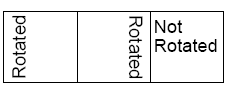# iTextSharp - Introducing Tables

Tables will probably be one of the most used elements in PDFs generated from ASP.NET applications to provide the structure for documents such as orders and invoices. This overview is not an exhaustive examination of tables, but provides an introduction to working with them through iTextSharp, and builds on the previous articles in this iTextSharp series:

Working with tables using iTextSharp is not that difficult, especially as many of the property names are so similar or identical to their counterparts within CSS and HTML. There is more than one class in iTextSharp that can be used to create tables, so for the avoidance of doubt, I will be using the PdfPTable class, which is designed specifically for use within PDF documents. At its most simplest, here is how to create a table and add it to a document:

PdfPTable table = new PdfPTable(3);

PdfPCell cell = new PdfPCell(new Phrase("Header spanning 3 columns"));

cell.Colspan = 3;

cell.HorizontalAlignment = 1; //0=Left, 1=Centre, 2=Right

The PdfPTable object is instantiated as a three column table - the integer 3 being passed into the constructor. Cells can be added in a number of ways. The first cell is set as a PdfPCell object, which can take a Phrase object in one of its 7 constructors. The Colspan is set to 3, which means that the cell will occupy the full width of the table, just as in HTML. The horizontal position of the text within the cell is set using one of three possible values. All possible values are shown as a comment. Following that, two rows of cells are added using the AddCell() method and the table is finally committed to the currently open document.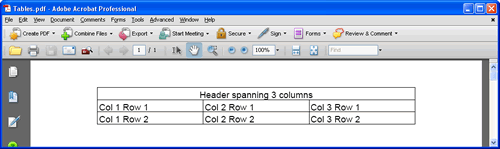The following effort queries a database, and presents the resulting data in a table. It also shows some other options that can be used for styling and presenting the table:

PdfPTable table = new PdfPTable(2);

//actual width of table in points

table.TotalWidth = 216f;

//fix the absolute width of the table

table.LockedWidth = true;

//relative col widths in proportions - 1/3 and 2/3

float[] widths = new float[] { 1f, 2f };

table.SetWidths(widths);

table.HorizontalAlignment = 0;

//leave a gap before and after the table

table.SpacingBefore = 20f;

table.SpacingAfter = 30f;

PdfPCell cell = new PdfPCell(new Phrase("Products"));

cell.Colspan = 2;

cell.Border = 0;

cell.HorizontalAlignment = 1;

string connect = "Server=.\\SQLEXPRESS;Database=Northwind;Trusted_Connection=True;";

using (SqlConnection conn = new SqlConnection(connect))

{

string query = "SELECT ProductID, ProductName FROM Products";

SqlCommand cmd = new SqlCommand(query, conn);

try

{

conn.Open();

{

{

}

}

}

catch(Exception ex)

{

Response.Write(ex.Message);

}

}

The table is initally created with 2 columns. Then the width of the table is set in points, and fixed. The width of the columns themselves are set relatively at one third and two thirds of the total table width. To set it a one fifth and 4 fifths, you would pass in 1f and 4f respectively. You can slo set the absolute widths by passing in values that together total the table width, for example:

float[] widths = new float[] { 100f, 116f };

A gap is created before and after the table by setting the SpacingBefore and SpacingAfter properties. This is useful if you have more than one table following on from another, as the default behaviour is to pin subsequent tables to the previous one, as in MS Word, where a quick tap of the Enter key has the same spacing effect. The border is removed from the first cell, which is treated as a header by setting the colspan to equal the number of columns in the table, and the text in the cell is centre-aligned, using the same value as that used for aligning the table in the document. Then the database is queried and the data returned in a SqlDataReader. As it is read, the data is consigned to cells which are added to the table: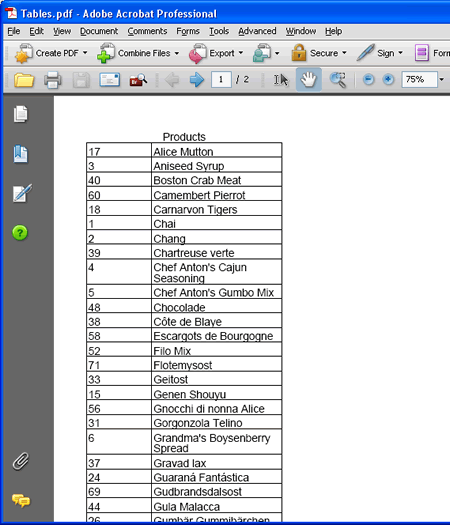The following snippet illustrates some of the options for formatting cells. As you will see, the creators of iTextSharp have followed the CSS names for properties as much as possible to make working with styling syntax as easy as possible (if you know your CSS, of course...)

PdfPTable table = new PdfPTable(3);

PdfPCell cell = new PdfPCell(new Phrase("Cell 2", new Font(Font.HELVETICA, 8f, Font.NORMAL, Color.YELLOW)));

cell.BackgroundColor = new Color(0, 150, 0);

cell.BorderColor = new Color(255,242,0);

cell.Border = Rectangle.BOTTOM_BORDER | Rectangle.TOP_BORDER;

cell.BorderWidthBottom = 3f;

cell.BorderWidthTop = 3f;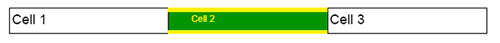We have seen on a number of occasions how a cell can stretch horizontally through the use of the Colspan property. But what about vertically? In HTML you would use the Rowspan property, but there is no equivalent in iTextSharp. So the answer is nested tables. The following code creates a four column table, with the bottom right cell stretching horizontally across three columns, and vertically by three rows. Well, that's the final appearance, but what actually happens is that a single column, three row table is nested within the bottom left cell. The cell that the table is nested within has its padding removed so that the table occupies all of the available space within it.

PdfPTable table = new PdfPTable(4);

table.TotalWidth = 400f;

table.LockedWidth = true;

PdfPTable nested = new PdfPTable(1);

PdfPCell nesthousing = new PdfPCell(nested);

PdfPCell bottom = new PdfPCell(new Phrase("bottom"));

bottom.Colspan = 3;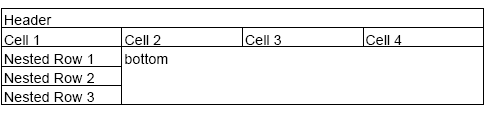Finally, in this look at tables, we see how the text content of a cell can be rotated (which is rather natty).

PdfPTable table = new PdfPTable(3);

table.TotalWidth = 144f;

table.LockedWidth = true;

table.HorizontalAlignment = 0;

PdfPCell left = new PdfPCell(new Paragraph("Rotated"));

left.Rotation = 90;

PdfPCell middle = new PdfPCell(new Paragraph("Rotated"));

middle.Rotation = -90;